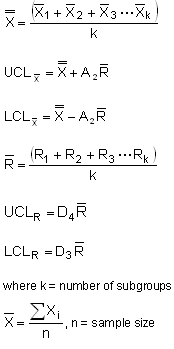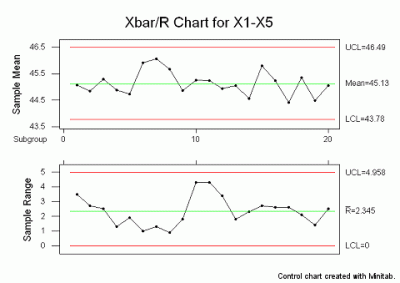## Control Chart Suggestion

Average And Range – X-Bar and R

## Control Chart Description

If the sample size is relatively small (e.g., less than 10-15), we can use the range instead of the standard deviation of a sample to construct control charts on X-Bar (arithmetic mean) and the range, R. The range of a sample is simply the difference between the largest and smallest observation. The X-bar and R control chart is used with continuous/variable data when subgroup or sample size is between 2 and 15.

## Control Chart FormulaeTable of Constants

## Control Chart Example